# B- Trees

## Introduction to B- Trees

A B – Tree can be defined as a multi-way search tree of order m such that it follows the conditions stated:

• All the internal nodes (except the root node) have at least m/2 children and at most m children.
• The non – leaf root node may have at most m non empty child and at least two child nodes.
• In a B- Tree, the root node is the only node that can exist without no child nodes.
• If a node has m children then it must have (m – 1) values. All these values of a particular node are in increasing order.
• All the values of a node that appear on the leftmost child of the node are smaller than the first value of that node. Similarly, all the values that appear on the rightmost child of a node are greater than the last value of that node.
• If a and b are any two ith and (i+1)th values of the node , where x < y, then all the values appearing on the (i+1)th sub tree of the that node are greater than x and less than y.
• All the leaf nodes should appear on the same level.
• The primary goal to use a B-tree is to reduce the number of disk access. Majority of tree operations such as searching, traversal ,insertion, etc, take O(n) time, where n is the height of the tree.
• A B-Tree of  degree T can have –
•  T-1 minimum keys.
• 2T-1 maximum keys.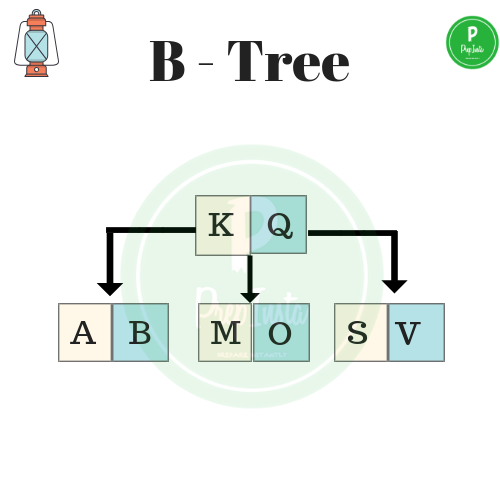• Insertion.
• Deletion

## Representation of B- Tree

The structure of a node in B-Tree can be represented as follows:

• struct btree_node
• {
•         int c;
•         int val[M + 1];
•         struct btree_node *child[M + 1]
• };
1. Here represents the number of children a particular node can have.
2. Array val represents the values of the node stored in it.
3. Array child stores the address of child nodes.
4. The macro M signifies the maximum number of values a node can hold.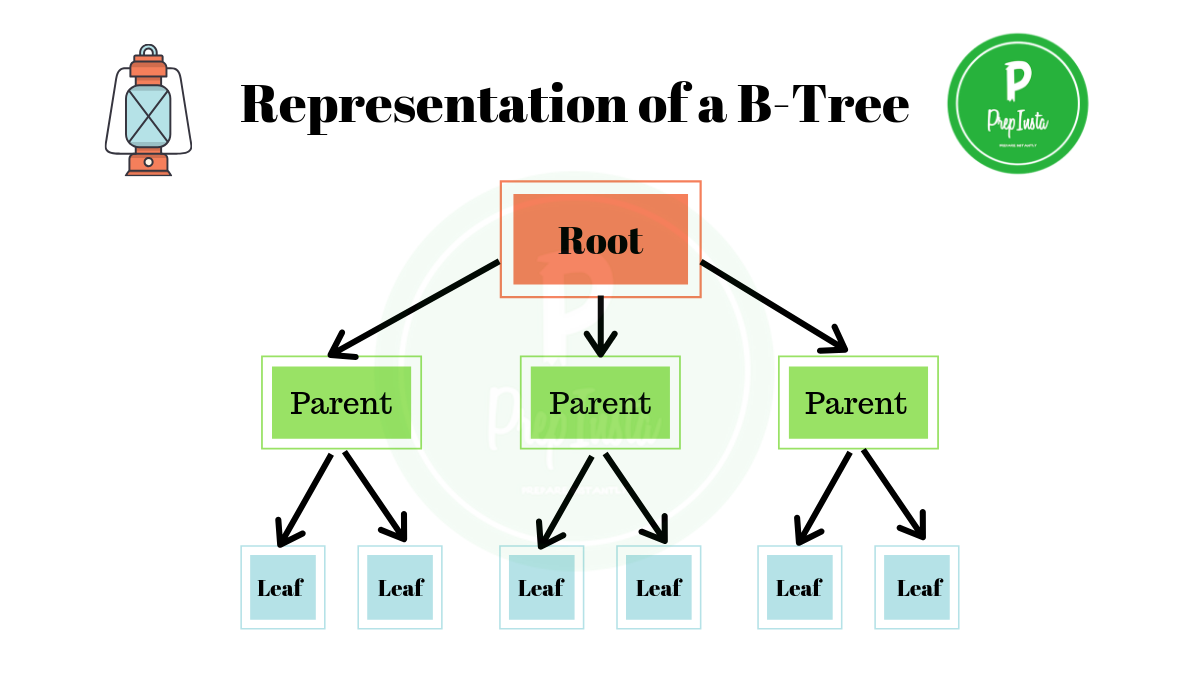## Creation of a B-Tree:

Let us consider a B-Tree of order 5. It means we can have a maximum number of 4 values that any node can hold.

For reference we see the given image. we can see that any node can have at most 4 values.

But if in any case we are given the degree of a tree; for instance consider t = 2, then,

• Minimum number of keys that a tree can hold can be calculated as
• min_key = t – 1=> 2 – 1 => min_key = 1
• Maximum number of keys that a tree can hold can be calculated as
• max_key = 2t – 1 => 2(2) – 1 => 4 – 1 => max_key = 3

Let us perform a step by step construction of a B-Tree.
Consider we have to construct a B-tree where the elements are given as, 20 , 30 , 35 , 85 , 5 , 10 , 40 , 55 , 60 , 38 , 25 , 7 , 78.

#### Step 1:

The first three elements  20 , 30 , 35 are added to a node.#### Step 2:

The element 85 is added to the node. Now if we jump back to the previous section we can see that a B-tree with degree t=2 can have at most 3 key values. Thus the node is split from the median.

Note: How to calculate median for splitting the node-

• Let x be a given key values in a node.
• n be the length of the node .
• Meadian = n[x] / 2.

Hence here we can see the length n[x] = 4.

Thus, Median =  n[x] / 2  => 4 / 2 = 2.

This means that the node will be split from the second key of the node.#### Step 3:

As the node is split  30 becomes the new root node of the tree.#### Step 4:

The element 5 is inserted.#### Step 5:

The element 10 is inserted.#### Step 6:

The element 40 is inserted#### Step 7:

The element 55 is added to the node.#### Step 8:

• To conserve the degree of the tree, we need to split the node where 55 was added. To do so we calculate the median which comes out to be 2.
• The node is split from the 2nd key. 40 moves upwards to the root node.
• The tree structure is shown as follows.#### Step 9:

The elements 38 and 60 are added to the B-Tree.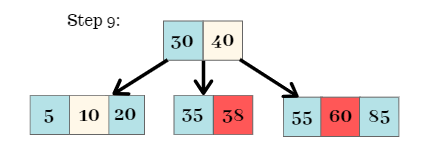#### Step 10:

• The element 25 is added to the node. The node has to split as each node in this tree can have at most 3 keys.
• The node is split from the Median, i.e, 2.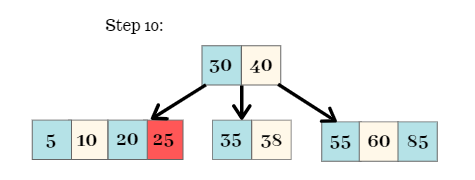#### Step 11:

The node is split from the 2nd key. Thus, 10 moves upwards to the parent / root node.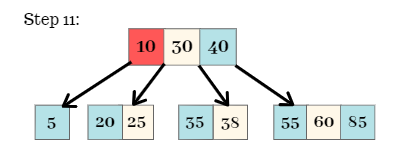#### Step 12:

The elements and 78 are added to the B-Tree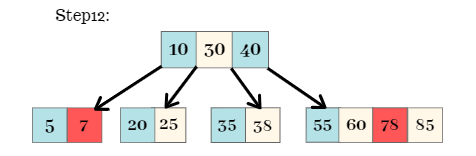#### Step 13:

• As the node is split the element 60 moves upward towards the parent / root node.
• Now, again we can see that the root node is to be split because each node can hold at most 3 keys.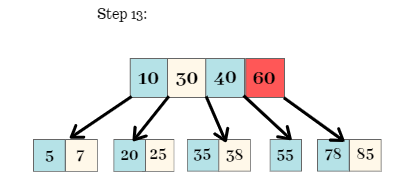#### Step 14:

• Again, we calculate the median of the node. The median can be calculated as follows,
• Median = n[x]  /  2.
• Median = 4 / 2.
• Median = 2.
• The node is split from the 2nd key which moves upwards and becomes the new root of the tree. The tree structure that follows is shown as follows,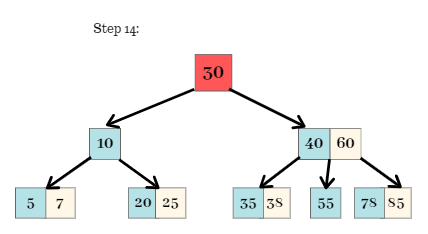• The final B-Tree after the concurrent insertion and splitting is shown as follows.
• This B-Tree fulfills all the properties of a B-Tree.
• Each node has either at least 1 key or at most 3 keys.
• Any node with number of keys exceeding the given number is split and re-arranged accordingly.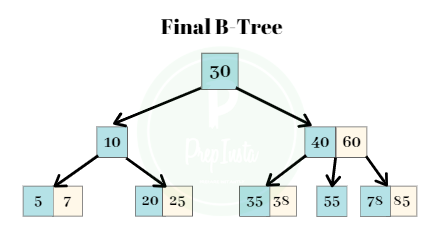### One comment on “B- Trees”

•Deepak

Thanks for perfect examples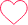0